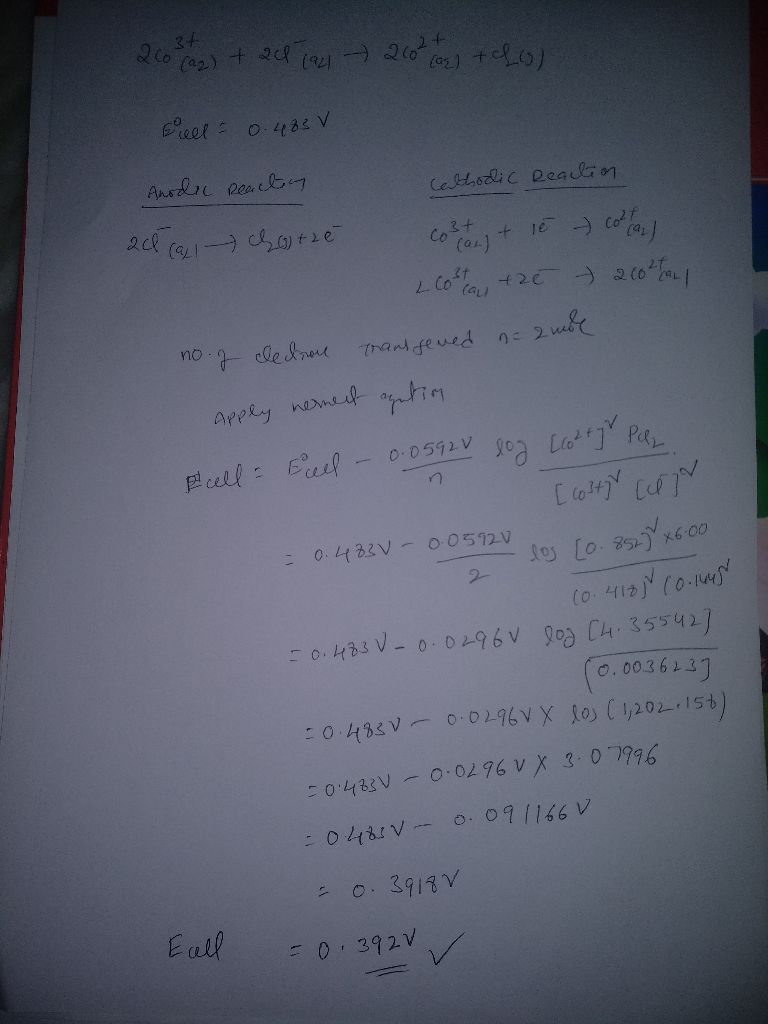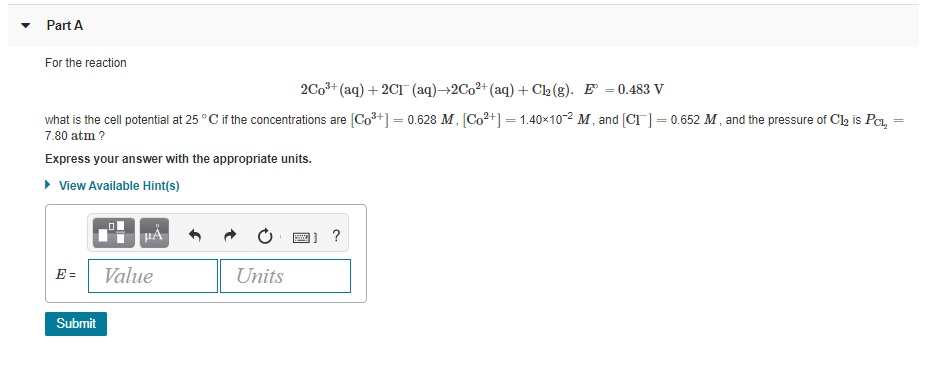Question

For the reaction 2Co3+(aq)+2Cl−(aq)→2Co2+(aq)+Cl2(g). E∘=0.483 V what is the cell potential at 25 ∘C if the concentrations are [Co3+]= 0.418 M , [Co2+]= 0.852 M , and [Cl−]= 0.144 M , and the pressure of Cl2 is PCl2= 6.00 atm ?#### Earn Coins

Coins can be redeemed for fabulous gifts.

Similar Homework Help Questions
• ### For the reaction 2Co3+(aq)+2Cl−(aq)→2Co2+(aq)+Cl2(g). E∘=0.483 V what is the cell potential at 25 ∘C if the...

For the reaction 2Co3+(aq)+2Cl−(aq)→2Co2+(aq)+Cl2(g). E∘=0.483 V what is the cell potential at 25 ∘C if the concentrations are [Co3+]= 0.815 M , [Co2+]= 0.498 M , and [Cl−]= 0.483 M , and the pressure of Cl2 is PCl2= 3.90 atm ? E=?

• ### For the reaction 2Co3+(aq)+2Cl−(aq)→2Co2+(aq)+Cl2(g). E∘=0.483 V what is the cell potential at 25 ∘C if the...

For the reaction 2Co3+(aq)+2Cl−(aq)→2Co2+(aq)+Cl2(g). E∘=0.483 V what is the cell potential at 25 ∘C if the concentrations are [Co3+]= 0.614 M , [Co2+]= 0.508 M , and [Cl−]= 0.321 M , and the pressure of Cl2 is PCl2= 7.90 atm ?

• ### For the reaction 2Co3+(aq)+2Cl−(aq)→2Co2+(aq)+Cl2(g). E∘=0.483 V what is the cell potential at 25 ∘C if the...

For the reaction 2Co3+(aq)+2Cl−(aq)→2Co2+(aq)+Cl2(g). E∘=0.483 V what is the cell potential at 25 ∘C if the concentrations are [Co3+]= 0.102 M, [Co2+]= 0.621 M, and [Cl−]= 0.307 M, and the pressure of Cl2 PCl2= 7.30 atm?

• ### For the reaction 2Co3+(aq)+2Cl−(aq)→2Co2+(aq)+Cl2(g). E∘=0.483 V what is the cell potential at 25 ∘C if the...

For the reaction 2Co3+(aq)+2Cl−(aq)→2Co2+(aq)+Cl2(g). E∘=0.483 V what is the cell potential at 25 ∘C if the concentrations are [Co3+]= 0.324 M , [Co2+]= 0.127 M , and [Cl−]= 0.680 M , and the pressure of Cl2 is PCl2= 6.50 atm ?

• ### For the reaction 2Co3+(aq)+2Cl−(aq)→2Co2+(aq)+Cl2(g). E∘=0.483 V what is the cell potential at 25 ∘C if the...

For the reaction 2Co3+(aq)+2Cl−(aq)→2Co2+(aq)+Cl2(g). E∘=0.483 V what is the cell potential at 25 ∘C if the concentrations are [Co3+]= 9.60×10−2 M , [Co2+]= 0.759 M , and [Cl−]= 0.755 M , and the pressure of Cl2 is PCl2= 5.90 atm?

• ### For the reaction 2Co3+(aq)+2Cl−(aq)→2Co2+(aq)+Cl2(g).  E∘=0.483 V what is the cell potential at 25 ∘C if the concentrations...

For the reaction 2Co3+(aq)+2Cl−(aq)→2Co2+(aq)+Cl2(g).  E∘=0.483 V what is the cell potential at 25 ∘C if the concentrations are [Co3+]= 0.594 M , [Co2+]= 0.858 M , and [Cl−]= 0.723 M , and the pressure of Cl2 is PCl2= 8.00 atm ?

• ### For the reaction 2Co3+(aq)+2Cl−(aq)→2Co2+(aq)+Cl2(g). E∘=0.483 V what is the cell potential at 25 ∘C if the...For the reaction 2Co3+(aq)+2Cl−(aq)→2Co2+(aq)+Cl2(g). E∘=0.483 V what is the cell potential at 25 ∘C if the concentrations are [Co3+]= 0.628 M , [Co2+]= 1.40×10−2 M , and [Cl−]= 0.652 M , and the pressure of Cl2 is PCl2= 7.80 atm ? Express your answer with the appropriate units. - Part A For the reaction 2CO3+ (aq) + 2CT (aq)+2Co2+ (aq) + Cl2(g). E=0.483 V what is the cell potential at 25°C if the concentrations are Co3+1 = 0.628 M, Co2+1...

• ### For the reaction 2Co3+(aq)+2Cl−(aq)→2Co2+(aq)+Cl2(g). E∘=0.483 V what is the cell potential at 25 ∘C if the...

For the reaction 2Co3+(aq)+2Cl−(aq)→2Co2+(aq)+Cl2(g). E∘=0.483 V what is the cell potential at 25 ∘C if the concentrations are [Co3+]= 8.10×10−2 M , [Co2+]= 0.419 M , and [Cl−]= 0.685 M , and the pressure of Cl2 is PCl2= 4.00 atm ? Express your answer with the appropriate units. E= ?

• ### for the reaction 2Co3+(aq)+2Cl−(aq)→2Co2+(aq)+Cl2(g).  E∘=0.483 V what is the cell potential at 25 ∘C if the concentrations...

for the reaction 2Co3+(aq)+2Cl−(aq)→2Co2+(aq)+Cl2(g).  E∘=0.483 V what is the cell potential at 25 ∘C if the concentrations are [Co3+]= 0.788 M , [Co2+]= 0.157 M , and [Cl−]= 0.702 M , and the pressure of Cl2 is PCl2= 2.90 atm ? Express your answer with the appropriate units.

• ### For the reaction 2Co3+(aq)+2Cl−(aq)→2Co2+(aq)+Cl2(g).  E∘=0.483 V what is the cell potential at 25 ∘C if the concentrations...

For the reaction 2Co3+(aq)+2Cl−(aq)→2Co2+(aq)+Cl2(g).  E∘=0.483 V what is the cell potential at 25 ∘C if the concentrations are [Co3+]= 0.223 M , [Co2+]= 0.551 M , and [Cl−]= 0.209 M , and the pressure of Cl2 is PCl2= 8.90 atm ? Express your answer with the appropriate units.